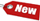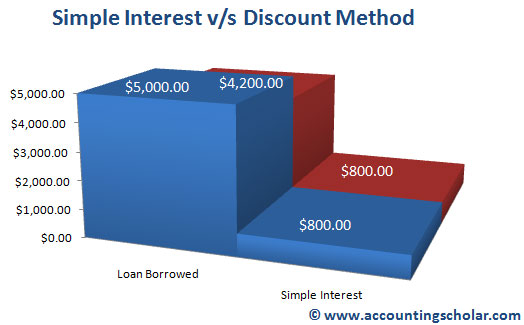Browse Accounting Lessons HereAccounting Terms & DefinitionsAccounting for Merchandising ActivitiesDebits and Credits (Double Entry Accounting)Business Valuation FormulasTime Value of Money & Present/Future ValuesComplex Debt & Equity InstrumentsCommon Stock & Shareholder's EquityAccounting & Finance RatiosValuing Common StockCorporate Income TaxesLower of Cost or Market (LCM) & Inventory ValuationChart of Accounts & BookkeepingBonds Payable & Long Term LiabilitiesCapital AssetsGAAP, Accrual & Cash Accounting, Information Commodity, Internal Controls & MaterialityWhat category of browser are you on this website? Accounting student (homework help) Finance professor (university research) Accounting manager (at work) Other Explore Careers in Accounting and FinanceVisit our section on Careers in Accounting & Finance to explore vast opportunities in this industry.

Annual Percentage Rate (APR) - True Measure of Interest Fees Charged by Credit Card Companies

Annual percentage rate (APR) is a true measure of the interest fees charged by credit card companies & banks. Annual percentage rate (APR) is the effective cost of credit which is the ratio of finance charges to the average amount of credit used in the life of the loan; this is expressed as a percentage per year. In this tutorial, we look at the calculation of APR for single payment loans & multiple instalment loans.

Single Payment Loans

A single payment loan is repaid in full on the maturity date and there are two ways of calculating APR on single loan payments: I) simple interest method and ii) the discount method. The difference between the simple interest method & the discount method is what the borrower actually receives in the form of a loan.

i) Simple Interest Method

Under the simple interest method, interest is calculated on the full original amount borrowed. The formula for simple interest is:

 Simple interest = Principal x Rate x Time = p x r x t Annual Percentage Rate = Average annual finance charge / Loan amount borrowed

Note that under the simple interest method, the stated simple interest rate & the Annual percentage rate (APR) will be the same. As an example, consider Peter borrowed a single-payment loan for \$5,000 for 2 years, at an interest rate of 8%. The interest charge over the life of the loan (2 years) will be:

 Simple interest = Principal x Rate x Time = p x r x t Simple interest = \$5,000 x 8% x 2 years Simple interest = \$800

Based on this simple interest, what will the APR be?

 Annual Percentage Rate = Average annual finance charge / Loan amount borrowed Annual Percentage Rate = \$400 / \$5,000 Annual Percentage Rate = 8%

2) Discount Method

Under the discount method, the loan borrower tends to pre-pay all the finance charges, after which interest is determined & deducted from the amount of the loan. The discount method always gives a higher APR than the simple interest method at the same interest rate because it deducts the total finance charge (total interest paid) from the amount of the loan borrowed. Therefore:

 Loan amount actually received from (1) = Loan amount – Finance Charge Loan amount actually received from (1) = \$5,000 - \$800 Loan amount actually received from (1) = \$4,200

From this calculation, we are deducting the total finance charge of \$800 from the loan amount borrowed of \$5,000 to arrive at the real loan amount borrowed, \$4,200.

 Annual Percentage Rate = Average annual finance charge / Loan amount borrowed Annual Percentage Rate = \$400 / \$4,200 Annual Percentage Rate = 9.52%

We see that the annual percentage rate is actually 9.52% and not the finance charge of 8% as we derived using the simple interest method. Thus, lenders are required to quote 9.52% interest on the loan, and not 8%.This graph shows the effects of the APR of 8% on the \$5,000 borrowed principal balance; total simple interest comes out to \$800 over a period of 2 years.